ISEE Middle Level Math : Data Analysis

Example Questions

Example Question #19 : Mode

Find the mode in this set of numbers: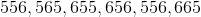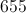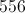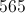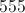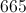Explanation:

Identify the most recurring number: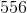Answer: The mode is 556.

Example Question #14 : Mode

Find the mode in this set of numbers;

11, 16, 14, 13, 12, 18, 14, 12, 14, 17, 15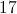Explanation:

The mode is the number that appears most frequently.

Answer: The mode is 14.

Example Question #21 : Mode

Find the mode in this set of numbers:

787, 778, 878, 877, 778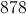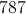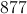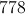Explanation:

The mode is the number that appears most frequently.

Answer: The mode is 778.

Example Question #22 : Mode

FInd the mode in this set of numbers:

22, 20, 22, 25, 21, 20, 12, 23, 20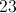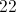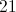Explanation:

The mode is the number that appears most frequently.

Answer: The mode is 20.

Example Question #23 : Mode

Find the mode in this set of numbers: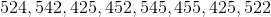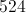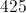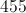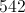Explanation:

The mode is the number that appears most frequently: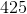Example Question #24 : Mode

Find the mode of this set of numbers: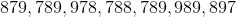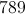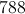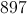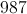Explanation:

The mode is the number that appears most frequently: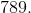Example Question #23 : Mode

Find the mode of this set of numbers: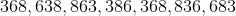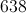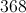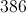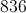Explanation:

The mode is the number that appears most frequently: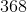Answer: The mode is 368.

Example Question #26 : Mode

Find the mode of this set of numbers: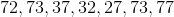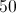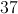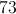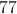Explanation:

The mode is the number that appears most frequently: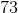Example Question #27 : Mode

Find the mode of this set of numbers: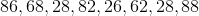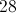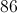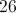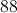Explanation:

The mode is the number that appears most frequently in the set:Answer: The mode is.

Example Question #28 : Mode

Find the mode of this set of numbers: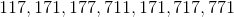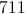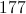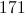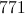Explanation:

The mode is the number that appears most frequently: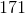All ISEE Middle Level Math Resources